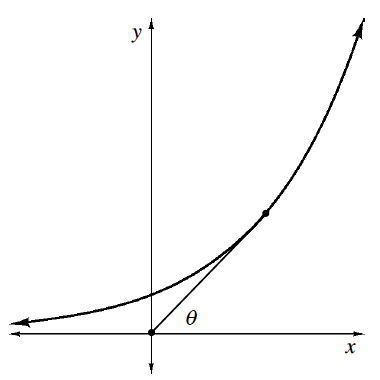### Home > APCALC > Chapter 7 > Lesson 7.4.4 > Problem7-219

7-219.

A particle moves along the curve $y = 2^x$ so that its $x$-coordinate increases at the constant rate of $5$ units per second. How fast is its angle of inclination $θ$ changing when $x = 2$?

(As shown in the diagram at right, $θ$ is the angle between the segment joining the point to the origin and the $x$-axis.)

$\tan(\theta) =\frac{\text{opposite side}}{\text{adjacent side}}$

What are the opposite and adjacent sides here?

$\tan(\theta) =\frac{y}{x}=\frac{2^{x}}{x}$

To obtain $θ$, use implicit differentiation in terms of $t$, since the $x$-coordinate and $θ$ are changing over time.